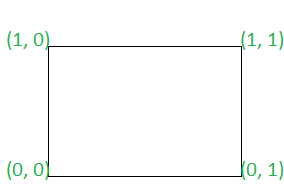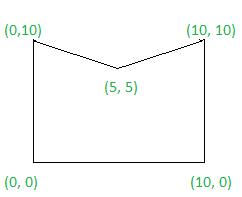Check if given polygon is a convex polygon or not

• Difficulty Level : Medium
• Last Updated : 09 Aug, 2021

Given a 2D array point[][] with each row of the form {X, Y}, representing the co-ordinates of a polygon in either clockwise or counterclockwise sequence, the task is to check if the polygon is a convex polygon or not. If found to be true, then print “Yes” . Otherwise, print “No”.

In a convex polygon, all interior angles are less than or equal to 180 degrees

Examples:

Input: arr[] = { (0, 0), (0, 1), (1, 1), (1, 0) }
Output: Yes
Explanation:Since all interior angles of the polygon are less than 180 degrees. Therefore, the required output is Yes.

Input : arr[] = {(0, 0), (0, 10), (5, 5), (10, 10), (10, 0)}
Output : No
Explanation:Since all interior angles of the polygon are not less than 180 degrees. Therefore, the required output is No.

Approach: Follow the steps below to solve the problem:

• Traverse the array and check if direction of cross product of any two adjacent sides of the polygon are same or not. If found to be true, then print “Yes”.
• Otherwise, print “No”

Below is the implementation of the above approach:

C++

 // C++ program to implement// the above approach #include using namespace std; // Utility function to find cross product// of two vectorsint CrossProduct(vector >& A){    // Stores coefficient of X    // direction of vector AA    int X1 = (A - A);     // Stores coefficient of Y    // direction of vector AA    int Y1 = (A - A);     // Stores coefficient of X    // direction of vector AA    int X2 = (A - A);     // Stores coefficient of Y    // direction of vector AA    int Y2 = (A - A);     // Return cross product    return (X1 * Y2 - Y1 * X2);} // Function to check if the polygon is// convex polygon or notbool isConvex(vector >& points){    // Stores count of    // edges in polygon    int N = points.size();     // Stores direction of cross product    // of previous traversed edges    int prev = 0;     // Stores direction of cross product    // of current traversed edges    int curr = 0;     // Traverse the array    for (int i = 0; i < N; i++) {         // Stores three adjacent edges        // of the polygon        vector > temp            = { points[i],                points[(i + 1) % N],                points[(i + 2) % N] };         // Update curr        curr = CrossProduct(temp);         // If curr is not equal to 0        if (curr != 0) {             // If direction of cross product of            // all adjacent edges are not same            if (curr * prev < 0) {                return false;            }            else {                // Update curr                prev = curr;            }        }    }    return true;} // Driver codeint main(){    vector > points        = { { 0, 0 }, { 0, 1 },            { 1, 1 }, { 1, 0 } };     if (isConvex(points)) {        cout << "Yes"             << "\n";    }    else {        cout << "No"             << "\n";    }}

Java

 // Java program to implement// the above approachclass GFG{   // Utility function to find cross product// of two vectorsstatic int CrossProduct(int A[][]){    // Stores coefficient of X    // direction of vector AA    int X1 = (A - A);     // Stores coefficient of Y    // direction of vector AA    int Y1 = (A - A);     // Stores coefficient of X    // direction of vector AA    int X2 = (A - A);     // Stores coefficient of Y    // direction of vector AA    int Y2 = (A - A);     // Return cross product    return (X1 * Y2 - Y1 * X2);} // Function to check if the polygon is// convex polygon or notstatic boolean isConvex(int points[][]){    // Stores count of    // edges in polygon    int N = points.length;     // Stores direction of cross product    // of previous traversed edges    int prev = 0;     // Stores direction of cross product    // of current traversed edges    int curr = 0;     // Traverse the array    for (int i = 0; i < N; i++) {         // Stores three adjacent edges        // of the polygon        int temp[][]= { points[i],                points[(i + 1) % N],                points[(i + 2) % N] };         // Update curr        curr = CrossProduct(temp);         // If curr is not equal to 0        if (curr != 0) {             // If direction of cross product of            // all adjacent edges are not same            if (curr * prev < 0) {                return false;            }            else {                // Update curr                prev = curr;            }        }    }    return true;} // Driver codepublic static void main(String [] args){    int points[][] = { { 0, 0 }, { 0, 1 },            { 1, 1 }, { 1, 0 } };     if (isConvex(points))    {        System.out.println("Yes");    }    else    {        System.out.println("No");    }}} // This code is contributed by chitranayal

Python3

 # Python3 program to implement# the above approach # Utility function to find cross product# of two vectorsdef CrossProduct(A):         # Stores coefficient of X    # direction of vector AA    X1 = (A - A)     # Stores coefficient of Y    # direction of vector AA    Y1 = (A - A)     # Stores coefficient of X    # direction of vector AA    X2 = (A - A)     # Stores coefficient of Y    # direction of vector AA    Y2 = (A - A)     # Return cross product    return (X1 * Y2 - Y1 * X2) # Function to check if the polygon is# convex polygon or notdef isConvex(points):         # Stores count of    # edges in polygon    N = len(points)     # Stores direction of cross product    # of previous traversed edges    prev = 0     # Stores direction of cross product    # of current traversed edges    curr = 0     # Traverse the array    for i in range(N):                 # Stores three adjacent edges        # of the polygon        temp = [points[i], points[(i + 1) % N],                           points[(i + 2) % N]]         # Update curr        curr = CrossProduct(temp)         # If curr is not equal to 0        if (curr != 0):                         # If direction of cross product of            # all adjacent edges are not same            if (curr * prev < 0):                return False            else:                                 # Update curr                prev = curr     return True # Driver codeif __name__ == '__main__':         points = [ [ 0, 0 ], [ 0, 1 ],               [ 1, 1 ], [ 1, 0 ] ]     if (isConvex(points)):        print("Yes")    else:        print("No") # This code is contributed by SURENDRA_GANGWAR

C#

 // C# program to implement// the above approachusing System; class GFG{   // Utility function to find cross product// of two vectorsstatic int CrossProduct(int [,]A){    // Stores coefficient of X    // direction of vector AA    int X1 = (A[1, 0] - A[0, 0]);     // Stores coefficient of Y    // direction of vector AA    int Y1 = (A[1, 1] - A[0, 1]);     // Stores coefficient of X    // direction of vector AA    int X2 = (A[2, 0] - A[0, 0]);     // Stores coefficient of Y    // direction of vector AA    int Y2 = (A[2, 1] - A[0, 1]);     // Return cross product    return (X1 * Y2 - Y1 * X2);} // Function to check if the polygon is// convex polygon or notstatic bool isConvex(int [,]points){    // Stores count of    // edges in polygon    int N = points.GetLength(0);     // Stores direction of cross product    // of previous traversed edges    int prev = 0;     // Stores direction of cross product    // of current traversed edges    int curr = 0;     // Traverse the array    for (int i = 0; i < N; i++) {         // Stores three adjacent edges        // of the polygon        int []temp1 = GetRow(points, i);        int []temp2 = GetRow(points, (i + 1) % N);        int []temp3 = GetRow(points, (i + 2) % N);        int [,]temp = new int[points.GetLength(0),points.GetLength(1)];        temp = newTempIn(points, temp1, temp2, temp3);         // Update curr        curr = CrossProduct(temp);         // If curr is not equal to 0        if (curr != 0) {             // If direction of cross product of            // all adjacent edges are not same            if (curr * prev < 0) {                return false;            }            else {                // Update curr                prev = curr;            }        }    }    return true;}public static int[] GetRow(int[,] matrix, int row)  {    var rowLength = matrix.GetLength(1);    var rowVector = new int[rowLength];     for (var i = 0; i < rowLength; i++)      rowVector[i] = matrix[row, i];     return rowVector;  }    public static int[,] newTempIn(int[,] points, int []row1,int []row2, int []row3)  {    int [,]temp= new int[points.GetLength(0), points.GetLength(1)];     for (var i = 0; i < row1.Length; i++){          temp[0, i] = row1[i];        temp[1, i] = row2[i];        temp[2, i] = row3[i];    }    return temp;  }          // Driver codepublic static void Main(String [] args){    int [,]points = { { 0, 0 }, { 0, 1 },            { 1, 1 }, { 1, 0 } };     if (isConvex(points))    {        Console.WriteLine("Yes");    }    else    {        Console.WriteLine("No");    }}} // This code is contributed by 29AjayKumar

Javascript


Output:
Yes

Time Complexity: O(N)
Auxiliary Space:O(1)

My Personal Notes arrow_drop_up